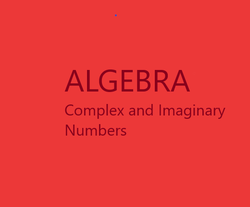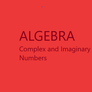# The Retired Professor Simplifies Complex and Imaginary Numbers

## This is a free to use algebra resource. It is designed to make it easy to understand complex and imaginary numbers for students and those assisting students with learning.

Complex numbers are important for the material that will follow. As we look at solving quadratic equations, we often have complex numbers occurring as solutions. So, we must familiar ourselves with complex numbers.

Modern textbooks often refer to complex numbers as imaginary numbers, but that term is misleading. A number with only an imaginary part is an imaginary number, meaning the only term is the one with the value i. If there is a real number term as well it should be called a complex number.

### Operating on Complex and Imaginary Numbers

If a square root of a negative number is taken, we quickly run into a problem.  No real number when multiplied by itself gives a negative number.  So, a new number called i is created and defined to be the square root of -1.  If we square this number, i2, we get -1.  When we cube i we get -i, and when we raise i to the fourth power we get 1.  As powers of i increase we go through the cycle of these four values.

If we multiply i by a coefficient we get what is called an imaginary number.

The name is misleading, these are actual numbers, and are of great importance.

A number consisting of a real term and an imaginary term of the form a + bi is called a complex number.

Complex numbers can be graphed using two axes, the horizontal axis labeled x and the vertical axis labeled iy.  So, think of complex numbers as two-dimensional numbers.  It is as simple as that.

When we add, or subtract, complex numbers we add, or subtract, the real part and add, or subtract, the imaginary part.

3 + 4i is to be added to 5 + 2i.  Adding 3 and 5 we get 8, and 4 plus 2 gives 6.

The sum above is 8 + 6i.

If we multiply the numbers (3 + 4i)(5 + 2i) we must treat the numbers as though they are binomials, and multiply as we would multiply binomials.

(3)(5) + (3)(2i) + (4i)(5) + (4i)(2i) = 15 + 6i + 20i + 8i2

Since i2 is -1, we get 15 + 6i + 20i – 8 = 7 + 26i

Definition:  The conjugate of a complex number has the opposite sign for the imaginary term.

The numbers 4 – 2i and 4 + 2i are conjugates of each other.

It is desirable to not have i in a denominator.  To fix the problem, multiply any complex number in the denominator by 1 in the form of its conjugate divided by itself.

As an example, start with the number below, and remove any imaginary number from its denominator.

(2 + 3i) ÷ (4 – 2i)

(2 + 3i) (4 + 2i) = 8 + 4i + 12i + 6i2 = 2 + 16i = 1 + 8i

(4 – 2i) (4 + 2i)    16 + 8i – 8i – 4i2        20            10

Notice the imaginary parts all disappear from the denominator.  This will always happen.

Absolute Value of a Complex Number

Finally, what is the absolute value of a complex number?  Well, the absolute value of a real number the distance from the point to the origin.  The same thing is true for absolute values of complex numbers.  Recall that complex numbers are two-dimensional, so we have an x distance and a y distance.  Since the axes are at right angles, the absolute value of a complex number requires the Pythagorean Theorem.

Find │3 – 4i│.

(32 + (-4)2)1/2 = (9 + 16)1/2 = 251/2 = 5

### Using a Graphing Calculator

Using a graphing calculator for complex numbers is easy.  The following is for the TI-83 and TI-84 calculators.

First, one must select a + bi under MODE.  Once that is done, look for the i key, it is a shift key.  Using the key allows you to enter a complex number.  When entering a complex number be certain to use parentheses, or an operation will only impact part of a complex number.  Use the operations +, -, *, and /, along with the absolute value command, abs(, as you would with real numbers.  Be certain to close the parentheses with ) once you finish entering a complex number in the absolute value command.  Your answer will be in complex number form.  This is a great tool for checking how accurate your work is, but do not substitute the calculator for actual needed practice.

There is also a conjugate command, which is hidden.  Most people will find the conjugate easy enough not to need it, but if you want to see it look under MATH and select the complex numbers.

### Other Parts of the Series

##### Early Chapters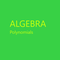This of a free resource where insights into algebra are provided to help both students and those helping students with algebra.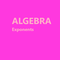This is a free resource on understanding algebra, and presented in a short presentation aimed at making things clear. It may help thoses studying algebra and those trying to help.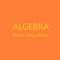This is the second part of a free resource to help with the basic understqanding of algebra. It can be used by students and those helping students witht the understanding.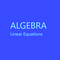Algebra simplified into a brief but thorough free resource can benefit both a parent helping a student and the student. This segment addresses linear equations.

This article contains links to affiliate programs and Adsense advertising.  These must use cookies to allow for proper crediting, and allow me to earn from qualifying purchases. As an Amazon Associate I earn from qualifying purchases.

Updated: 02/09/2021, blackspanielgallery
1

Only logged-in users are allowed to comment. Login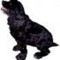blackspanielgallery on 02/12/2021

Use of calculators is here to help parents quickly check work, not as a resource for students. Math develops a logical way of thinking, not just finding solutions to problems, and overuse of calculators is not always a good idea. It gets the answer fastr, and when I write math for a publisher I use one to elimiante careless errors and speed things up to meet deadlines, but students need to be taught how to work things out, hence have the logic thought process involved.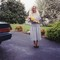DerdriuMarriner on 02/12/2021

blackspanielgallery, Thank you for the practical information and the product line.
Are complex and imaginary numbers taught through books and practice or books, calculator and practice? Some schools have kindergarten through second grade Monday through Friday and third through 12th grade just two days a week. Wouldn't teachers just about have to incorporate calculator instruction and use in such a virtual instruction-dependent situation?

#### You might also like

Collecting Seashells: Types of Shells

A look at some seashells you may see on Florida beaches with photos of my own...

Buttons from the Rag Bag! Button Jar Math Activities

Save the buttons from discarded clothing and recycle them as fun, hands-on ma...

Homeschooling Socialization: An Oxymoron?

Homeschooling & socialization is like peanut butter & old shoes, according to...

Disclosure: This page generates income for authors based on affiliate relationships with our partners, including Amazon, Google and others.